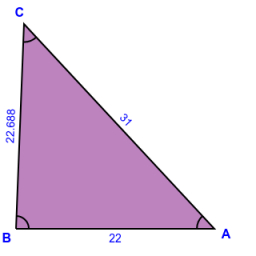# SAS calculation

Given the triangle ABC, if side b is 31 ft., side c is 22 ft., and angle A is 47°, find side a. Please round to one decimal.

a =  22.7 ft

### Step-by-step explanation:

Try calculation via our triangle calculator.Did you find an error or inaccuracy? Feel free to write us. Thank you!

Tips for related online calculators
The Pythagorean theorem is the base for the right triangle calculator.
Do you want to convert time units like minutes to seconds?
Cosine rule uses trigonometric SAS triangle calculator.